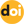The peer-reviewed scientific and technology journal. ISSN: 0204-3327

# Some Issues of Teaching the Dynamics of Nuclear Reactors

3/18/2021https://doi.org/10.26583/npe.2021.1.14

### UDC: 621.039.514.4:621.039.515:621.039.516.2:378

The article considers a number of methodological issues of teaching the dynamics of nuclear reactors in order to optimize curricula to achieve a closer relationship between courses in theoretical, experimental and computational physics of nuclear reactors by clarifying a number of formulations, simplifying mathematical constructions and rationalizing the conceptual apparatus. Particular attention is paid to the introduction of the basic concepts of the nuclear reactor dynamics, – reactivity, generation times and neutron lifetimes, – based on the simplest balance of generation rates and neutron loss in the fission process. The preference of using reactivity in the Λ-scale – r = ρ/Λ is shown. It is proposed to describe the nuclear reactor dynamics based on a modified form of the integral dynamics equation. In this form, the equation is specified only by the time dependence of reactivity and the integral kernel, i.e., the reproduction function of delayed neutron precursors (DNP). This approach unifies the consideration of direct and inverse problems of dynamics, reducing them to calculating the integral for reproducing the DNPs. In the presence of several fissile nuclides, the kernel of the integral equation is the sum of the corresponding reproduction functions, and the latter do not require generally to be represented by the sum of exponentials. This allows us not to specify the number of groups of delayed neutron emitters and not to introduce simplifying assumptions in situations that have traditionally been considered under the assumption of one group of delayed neutrons and one fissile nuclide. The proposed changes reduce the physical volume of educational materials, but retain their semantic content and allow for more hours in the curricula for in-depth study of a number of pressing issues related to the management and identification of multiplying systems of various types.

1. Kazansky Yu.A., Slekenichs Ya.V. Kinetics of Nuclear Reactors. Reactivity Coefficients. Introduction into Dynamics. Moscow. NIYaU MIFI Publ., 2012, 300 p. (in Russian).
2. Seleznev E.F. Kinetics of Fast Neutron Reactors. Moscow. Nauka Publ., 2013, 239 p. (in Russian).
3. Bahman Z. Neutronic Analysis for Nuclear Reactor Systems. Springer Publ., 2019, 672 p.
4. Marguet S. The Physics of Nuclear Reactors. Springer Publ., 2017, 1462 p.
5. Oka Y.; Suzuki K. Nuclear Reactor Kinetics and Plant Control. Springer Publ., 2013, 305 p. 6. Karnachuk V.I. Systems of Automatic Leveling of Neutron Flux in Nuclear Reactors. Tomsk. Tomsk Polytechnic University Publ., 2009. 221 p. (in Russian).
6. Halimonchuk V.A. Reactor Dynamics with Distributed Parameters in Investigations of Transient Operating Modes of VVER and RBMK. Kiev. Osnova Publ., 2008, 228 p. (in Russian).
7. Semenov V.K. Kinetics and Regulation of Nuclear Reactors. Ivanovo. Ivanovo State Power Engineering Institute Publ., 2009, 144 p. (in Russian).
8. Rumyantsev G.Ya., Toshinskiy G.I. Physics and Dynamics of Nuclear Reactors. Obninsk. IATE Publ., 2006, 202 p. (in Russian).
9. Ionov V.S. Distributed Neutron Dynamics of the VVER Cores. Moscow. Izdat Publ., 2005, 311 p. (in Russian).
10. Akhiezer A.I., Pomeranchuk I.Ya. Introduction in the Theory of Neutron Multiplying Systems (Reactors). Moscow. IzDAT Publ., 2002, 368 p. (in Russian).
11. Yurkevich G.P. Control Systems for Power Reactors. Moscow. ELEKS-KM Publ., 2001, 344 p. (in Russian).
12. Ilchenko A.G. Transient and Unsteady Processes in Nuclear Reactors. Ivanovo. Ivanovo State Power Engineering Institute Publ., 2000, 116 p. (in Russian).
13. Kolesov V.F. Aperiodic Impulse Reactors. Moscow. RFYaTs-VNIIEF Publ., 1999, 1032 p. (in Russian).
14. Kolesov V.F., Leppik P.A., Pavlov S.P., Plyutinsky V.I., Sabaev E.F., Torlin B.Z., Shevelyov Ya.V. Dynamics of Nuclear Reactors. Moscow. Energoatomizdat Publ., 1990, 518 p. (in Russian).
15. Sarkisov A.A., Puchkov V.N. Physics of Transients in Nuclear Reactors. Moscow: Energoatomizdat Pibl., 1983, 264 p. (in Russian).
16. Bartolomey G.G., Bat G.A., Baibakov V.D., and Alkhutov M.S. Fundamentals of the Theory and Methods of Calculating Nuclear Power Reactors. Moscow. Energoizdat Publ., 1982, 512 p. (in Russian).
17. Emelyanov I.Ya., Efanov A.I., Konstantinov L.V. Scientific and Technical Fundamentals of Nuclear Reactor Control. Moscow. Energoizdat Publ., 1981, 360 p. (in Russian).
18. Lewins J. Nuclear Reactor Kinetics and Control. Pergamon Press, 1978, 275 p.
19. Goryachenko V.D. Methods for Studying the Stability of Nuclear Reactors. Moscow. Atomizdat Publ., 1977, 296 p. (in Russian).
20. Hetrick D.L. Dynamics of Nuclear Reactors. The University of Chicago Press, 1971, 542 p.
21. Akcasu Z., Lellouche G.S., Shotkin L.M. Mathematical Methods in Nuclear Reactor Dynamics. Academic Press, 1971, 465 p.
22. Keepin G.R. Physics of Nuclear Kinetics. Addison-Wesley Pub. Co, 1965, 435 p.
23. Ash M. Nuclear Reactor Kinetics. McGraw Hill, 1965, 415 p.
24. Soodak G., Campbell E. Elementary Pile Theory. Uspekhi Fizicheskikh Nauk. 1950, v. 42, pp. 93-156; DOI: https://doi.org/10.3367/UFNr.0042.195009g.0093 (in Russian).
25. Eiring G., Lin S.G., Lin S.M. Fundamentals of Chemical Kinetics. Moscow. Mir Publ., 1983, 528 p. (in Russian).
26. Mitenkov F.M., Bagdasarov Yu.E., Buksha Yu.K., Vostokov V.S., Gorbunov V.S., Zayets N.P., Samoilov O.B., Kuznetsov I.A., Kozyrev V.D., Ashurko Yu.M., Sheinkman A.G. Engineering methods of analysis of modes with natural circulation in BN type installations. Atomnaya Energiya. 1987, v. 62, iss. 3, pp. 147-154 (in Russian).
27. Yuferov A.G. Reactimeters and Reactivity Measurement. Certificate of State Registration of bibliographic database No. 2016620830, June 21, 2016 (in Russian).
28. Kolesov V.F. Origins of inaccuracies in reactivity determined by inverse solution of kinetic equations. Proceedings of RFYaTs:VNIIEF. 2016, no. 21, part 1, pp. 130-153 (in Russian).
29. Fokin A.B., Potapenko P.T. Methods for calculating the spatial kinetics of reactors. Atomnaya Tekhnika za Rubezhom. 1974, no. 12, pp. 3-9 (in Russian).
30. Yuferov A.G., Ibragimov R.L. Reactimeter as an Adaptive Digital Filter. Atomnaya Energiya. 2005, v. 98, iss. 4, pp. 253-260 (in Russian).
31. Yuferov A.G. Generalized Linear Model for Identification of Nuclear Reactor Kinetics Parameters. Atomnaya Energiya. 2010, v. 108, iss. 1, pp. 8-12 (in Russian).
32. Yuferov A.G. Quadrature Formulas for the Integral Equations of Kinetics and Digital Reactimeters. Izvestiya vuzov. Yadernaya Energetika. 2017, no. 2, pp. 93-105 (in Russian).